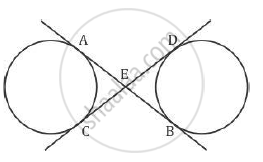Share

# common tangents AB and CD to the two circles with centres 01and 02 intersect at E. Prove that AB = CD. - CBSE Class 10 - Mathematics

ConceptCircles Examples and Solutions

#### Question

In Figure 1, common tangents AB and CD to the two circles with centres 01and 0intersect at E. Prove that AB = CD.#### SolutionGiven: AB and CD are common tangents to both the circles.
To prove: AB = CD
Proof:
We know that two tangents drawn to a circle for the same exterior point are
equal.
Thus we get
AE = EC (i)
Similarly
ED = EB (ii)
AB = AE + EB (iii)
and
CD = CE + ED (iv)
AB = EC + EB from (i) and (iii)
CD = EC + EB from (ii) and (iv)
Therefore AB = CD
Hence proved.

Is there an error in this question or solution?

#### Video TutorialsVIEW ALL 

Solution common tangents AB and CD to the two circles with centres 01and 02 intersect at E. Prove that AB = CD. Concept: Circles Examples and Solutions.
S